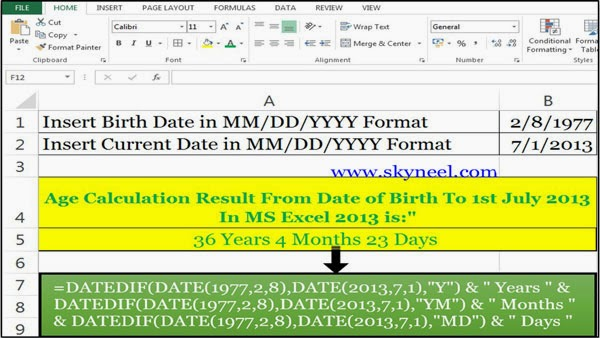# How to Age calculation From Date of Birth – MS EXCEL

Age calculation from date of birth to any specified date by Excel DATEDIF function. You can calculate number of year,month,days.

Hi guys, most of the time we need to know exact age but a question arise how to age calculation from date of birth. Today we talk how you can easily age calculation from date of birth to any specified date in excel. Excel’s DATEDIF function to calculate any present age of any person’s from the date of birth to any specific given date.

If you want to calculate current age of any person in number of years, months and the days from birth date then you have to use MS Excel’s most popular function DATEDIF(). For eg. If you want to find out number of years, months and the days of any person age from his date of birth to any specific date like 08 Feb, 1977 to 01st July, 2013.

### Steps for age calculation from date of birth :

Step 1. Prepare the following sheet in Microsoft Excel.

Step 2: Type following formula in cell A5 to find out the date of birth:=DATEDIF(DATE(1977,2,8), DATE(2013,7,1),”Y”) & ” Years ” & DATEDIF(DATE(1977,2,8), DATE(2013,7,1),”YM”) & ” Months ” & DATEDIF(DATE(1977,2,8), DATE(2013,7,1),”MD”) & ” Days “

Step 3: When you press enter key, Excel displays the result “36 Years 4 Months 23 days” in cell A5.

In this condition you have to feed DATEDIF function multiple times in a formula for  age calculation from date of birth. It easily calculate the number of years, months, and then the number of days. Type following formula’s given below in Cell C11, C12 & C13, to separate calculate of years months and days. The three parts to the formula are:### How to use Formula in Excel for age calculation from date of birth:

To Calculate Number of Years:
Cell C11 = DATEDIF(DATE(1977,2,8),DATE(2013,7,1),”Y”) & ” Years ”
To Calculate Number of Months:
Cell C12 =DATEDIF(DATE(1977,2,8),DATE(2013,7,1),”YM”) & ” Months ”
To Calculate Number of Days:
Cell C13 =DATEDIF(DATE(1977,2,8),DATE(2013,7,1),”MD”) & ” Days ”

### Concatenating the Formula Together

The ampersand operator (&) is used to combine or concatenation or join different data in a single formula. For example, the (&) operator is used with the DATEDIF function to join the different part of formula.

### The DATE ( ) Function

The DATE () function is used to return the number that represents the date in MS Office Excel date time code.<

I hope you like this post. Now, you will be able how to age calculation from date of birth. Kindly Give your important suggestion in the comment box and also share this article with your friends to show your knowledge in excel. Thanks.